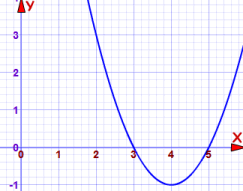# Zero Product Property

The "Zero Product Property" says that:

If   a × b = 0   then   a = 0   or   b = 0
(or both a=0 and b=0)

It can help us solve equations:

### Example: Solve (x−5)(x−3) = 0

The "Zero Product Property" says:

If  (x−5)(x−3) = 0  then   (x−5) = 0   or   (x−3) = 0

Now we just solve each of those:

For (x−5) = 0 we get x = 5

For (x−3) = 0 we get x = 3

And the solutions are:

x = 5, or x = 3

Here it is on a graph:y=0 when x=3 or x=5

## Standard Form of an Equation

Sometimes we can solve an equation by putting it into Standard Form and then using the Zero Product Property:

The "Standard Form" of an equation is:

(some expression) = 0

In other words, "= 0" is on the right, and everything else is on the left.

x2 − 7 = 0

## Standard Form and the Zero Product Property

So let's try it out:

### Example: Solve 5(x+3) = 5x(x+3)

It is tempting to divide by (x+3), but that is dividing by zero when x = −3

So instead we can use "Standard Form":

5(x+3) − 5x(x+3) = 0

Which can be simplified to:

(5−5x)(x+3) = 0

5(1−x)(x+3) = 0

Then the "Zero Product Property" says:

(1−x) = 0, or (x+3) = 0

And the solutions are:

x = 1, or x = −3

And another example:

### Example: Solve x3 = 25x

It is tempting to divide by x, but that is dividing by zero when x = 0

So let's use Standard Form and the Zero Product Property.

Bring all to the left hand side:

x3 − 25x = 0

Factor out x:

x(x2 − 25) = 0

x2 − 25 is a difference of squares, and can be factored into (x − 5)(x + 5):

x(x − 5)(x + 5) = 0

Now we can see three possible ways it could end up as zero:

x = 0, or x = 5, or x = −5

509, 510, 1140, 4030, 1141, 4031, 2292, 4032, 2293, 4033